# Surface Area And Volume Cube Cuboid Solution of TS & AP Board Class 8 Mathematics

#### Surface Area And Volume Cube Cuboid Solution of TS & AP Board Class 8 Mathematics

EXERCISE:-14.1

Question 1.

There are two cuboidal boxes as shown in the given figure. Which box requires the less amount of material to make?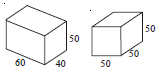Measurement of box 1

Length = 60 cm,

Height = 50 cm

Volume of cuboid = Length × Breadth × Height

= 60 × 40 × 20

= 12000 cm3

Measurement of box 2

Side = 50cm

Volume of cube = (side)3

= (50)3

= 50 × 50 × 50

= 125000 cm3

Box 1 requires less amount of material.

Question 2.

Find the side of a cube whose surface area is 600 cm2.

Let the side be S.

∴ surface area of cube = 6× side2

⇒ 600 = 6 × s2

⇒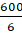= s2

⇒ s2 = 100

⇒ s = √100

⇒ s = 10 cm

Question 3.

Prameela painted the outer surface of a cabinet of measures 1m × 2m × 1.5m. Find the surface area she cover if she painted all except the bottom of the cabinet?

Measurement of the cuboid

Length(L) = 1 m breadth(B) = 2 m and height(H) = 1.5 m

Total surface area = 2(LB + BH + LH)

= 2(1 × 2 + 2 × 1.5 + 1 × 1.5)

= 2(2 + 3 + 1.5)

= 2(6.5)

= 13 cm2

Area of bottom surface = 1 × 2 = 2m2

Hence, area covered by painting = 13 – 2 = 11 m2

Question 4.

Find the cost of painting a cuboid of dimensions 20cm × 15 cm × 12 cm at the rate of 5 paisa per square centimeter.

Measurement of the cuboid

Length(L) = 20 cm breadth(B) = 15 cm and height(H) = 12cm

Total surface area = 2(LB + BH + LH)

= 2(20 × 15 + 15 × 12 + 20 × 12)

= 2(300 + 180 + 240)

= 2(720)

= 1440 cm2

Rate of painting 1cm2 = ₹0.05

Rate of painting a whole cuboid = 0.05 × 1440

= ₹72

EXERCISE:-14.2

Question 1.

Find the volume of the cuboid whose dimensions are given below.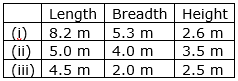i: Length = 8.2 m Breadth = 5.3m and Height = 2.6m

Volume of cuboid = Length × Breadth × Height

= 8.2 × 5.3 × 2.6

= 112.996 m3

ii: Length = 5.0 m Breadth = 4.0m and Height = 3.5m

Volume of cuboid = Length × Breadth × Height

= 5 × 4 × 3.5

= 70 m3

iii: Length = 4.5 m Breadth = 2m and Height = 2.5m

Volume of cuboid = Length × Breadth × Height

= 4.5 × 2 × 2.5

= 22.5 m3

Question 2.

Find the capacity of the tanks with the following internal dimensions. Express the capacity in cubic meters and liters for each tank.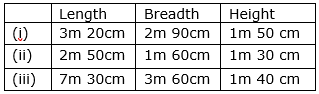i: Length = 3m 20cm = 3.2m

Breadth = 2m 90cm = 2.9m

Height = 1m 50cm = 1.5m

Volume of cuboid = Length × Breadth × Height

= 3.2 × 2.9 × 1.5

= 13.92 m3

ii: Length = 2m 50cm = 2.5m

Breadth = 1m 60cm = 1.6m

Height = 1m 30cm = 1.3m

Volume of cuboid = Length × Breadth × Height

= 2.5 × 1.6 × 1.3

= 5.2 m3

iii: Length = 7m 30cm = 7.3m

Breadth = 3m 60cm = 3.6m

Height = 1m 40cm = 1.4m

Volume of cuboid = Length × Breadth × Height

= 7.3 × 3.6 × 1.4

= 36.792 m3

Question 3.

What will happen to the volume of a cube if the length of its edge is reduced to half? Is the volume get reduced? If yes, how much?

Let x be the length of the cube

Volume of cube = (side)3

= x3

If the length of its edge is reduced to half, then length would be =Now, Volume of cube =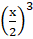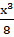Yes, the volume gets reduced bym3.

Question 4.

Find the volume of each of the cube whose sides are.

(i) 6.4 cm (ii) 1.3 m (iii) 1.6 m.

(i) Side = 6.4cm

Volume of cube = (side)3

= (6.4) 3

= 6.4 × 6.4 × 6.4

= 262.144 cm3

(ii) Side = 1.3cm

Volume of cube = (side)3

= (1.3) 3

= 1.3 × 1.3 × 1.3

= 2.197 m3

(iii) Side = 1.6m

Volume of cube = (side)3

= (1.6) 3

= 1.6 × 1.6 × 1.6

= 4.096 m3

Question 5.

How many bricks will be required to build a wall of 8 m long, 6m height and 22.5 cm thick, if each brick measures 25 cm by 11.25 cm by 6 cm?

Measurements of Wall:

Length = 800cm Breadth = 600cm and Height = 22.5cm

Volume of wall = Length × Breadth × Height

= 800 × 600 × 22.5

Measurements of Brick:

Length = 25cm Breadth = 11.25cm and Height = 6cm

Volume of wall = Length × Breadth × Height

= 25 × 11.25 × 6

Number of bricks required build a wall =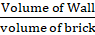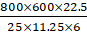= 32 × 100 × 2

= 64 × 100

= 6400

Hence, 6400 bricks are required to build the wall.

Question 6.

A cuboid is 25 cm long, 15 cm broad, and 8 cm high. How much of its volume will differ from that of a cube with the edge of 16 cm?

Measurement of cuboid

Length = 25 cm Breadth = 15 cm and Height = 8 cm

Volume of cuboid = Length × Breadth × Height

= 25 × 15 × 8

= 3000 cm3

Measurement of cube

Side = 16cm

Volume of cube = (side)3

= (16) 3

= 16 × 16 × 16

= 4096 cm3

Difference between the volumes = 4096 – 3000 cm3 = 1096 cm3

Volume of cuboid differs from volume of cube by 1096 cm3.

Question 7.

A closed box is made up of wood which is 1cm thick. The outer dimensions of the box is 5 cm × 4 cm × 7 cm. Find the volume of the wood used.

Measurement of box from outside:

Length = 5 cm Breadth = 4 cm and Height = 7 cm

Volume of cuboid = Length × Breadth × Height

= 5 cm × 4 cm × 7 cm

= 140 cm3

Measurement of box from the inside

Length = 4 cm

Height = 6 cm

Volume of cuboid = Length × Breadth × Height

= 4 cm × 3 cm × 6 cm

= 72 cm3

Volume of wood used = volume of the box from outside – volume of the box from inside

= 140 – 72

= 68 cm3

Question 8.

How many cubes of edge 4cm, each can be cut out from cuboid whose length, breadth and height are 20 cm, 18 cm and 16 cm respectively.

Measurement of cuboid

Length = 20 cm Breadth = 18 cm and Height = 16 cm

Volume of cuboid = Length × Breadth × Height

= 20 × 18 × 16

= 5760 cm3

Measurement of cube

Side = 4 cm

Volume of cube = (side)3

= (4) 3

= 4 × 4 × 4

= 64 cm3

Number of cube which can be cut from the cuboid =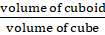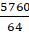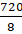= 90

90 cubes can be cut out from the cuboid.

Question 9.

How many cuboids of size 4 cm × 3 cm × 2 cm can be made from a cuboid of size 12 cm × 9 cm × 6 cm?

Measurement of bigger cuboid

Length = 12 cm Breadth = 9 cm and Height = 6 cm

Volume of cuboid = Length × Breadth × Height

= 12cm × 9cm × 6cm

Measurement of smaller cuboid

Length = 4 cm Breadth = 3 cm and Height = 2 cm

Volume of cuboid = Length × Breadth × Height

= 4cm × 3cm × 2cm

Number of smaller cuboids which can be made from bigger cuboid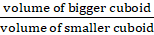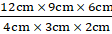= 3 × 3 × 3

= 27 cuboids

27 cuboids of smaller cuboids can be made from bigger cuboid.

Question 10.

A vessel in the shape of a cuboid is 30 cm long and 25 cm wide. What should be its height to hold 4.5 liters of water?

We know that,

1 liter is 1000cm3,

∴ 4.5 liters = 4.5 × 1000 = 4500 cm3

Measurement of vessel

Length = 30 cm,

And Height =?

Let the height be h cm.

We are given the capacity which this vessel can hold i.e. the volume of the vessel.

Volume of vessel = Length × Breadth × Height

4500 = 300 × 25 × h cm

4500 = 750 × h

h =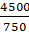h =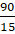h = 6 cm

Hence, height of the vessel is 6cm which can hold 4.5 liters.

## MATHS VIDEOS